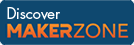Cody

# Problem 3. Find the sum of all the numbers of the input vector

Created by Cody Team in Community

Find the sum of all the numbers of the input vector x.

` Examples:`
```Input  x = [1 2 3 5]
Output y is 11
```
```Input  x = [42 -1]
Output y is 41
```

### Solution Stats

62.56% Correct | 37.44% Incorrect
Last solution submitted on Mar 26, 2019

#### TagsMATLAB and Simulink resources for Arduino, LEGO, and Raspberry Pi